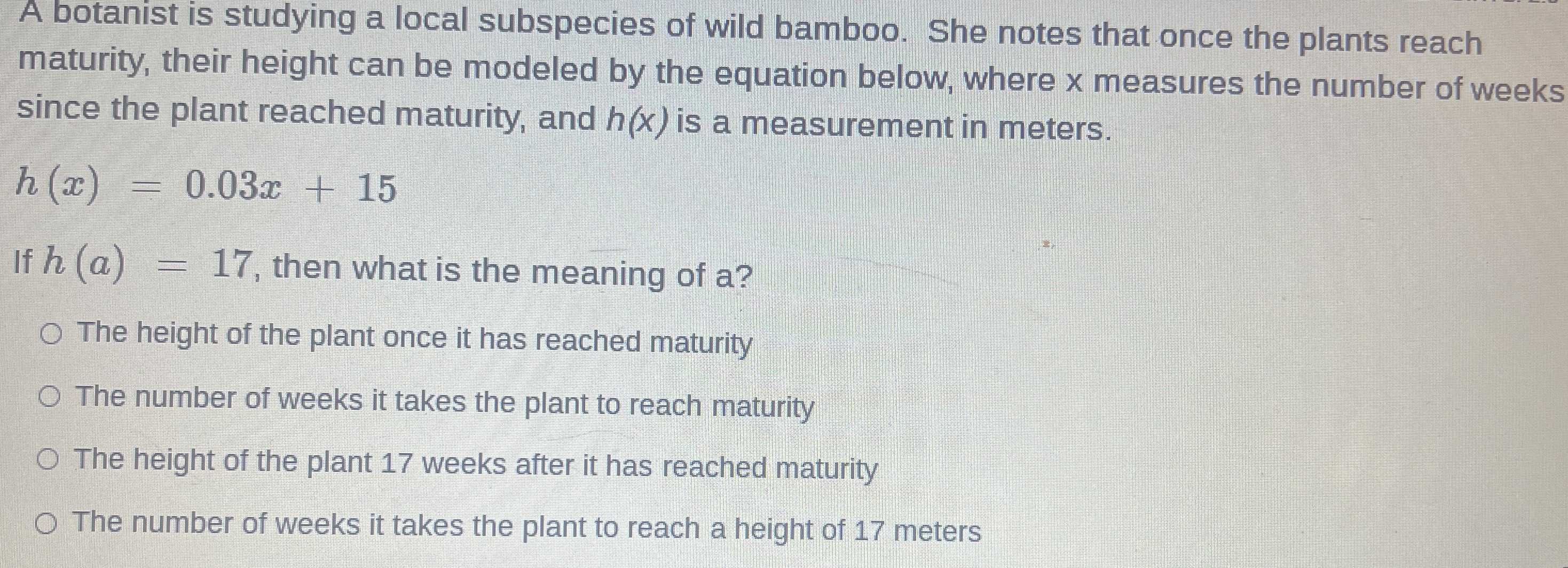### ¿Todavía tienes preguntas de matemáticas?

Pregunte a nuestros tutores expertos
Algebra
PreguntaA botanist is studying a local subspecies of wild bamboo. She notes that once the plants reach maturity, their height can be modeled by the equation below, where $$x$$ measures the number of weeks since the plant reached maturity, and $$h ( x )$$ is a measurement in meters.

$$h ( x ) = 0.03 x + 15$$

If $$h ( a ) = 17$$ , then what is the meaning of a?

(A)The height of the plant once it has reached maturity

(B)The number of weeks it takes the plant to reach maturity

(C)The height of the plant $$17$$ weeks after it has reached maturity

(D)The number of weeks it takes the plant to reach a height of $$17$$ meters